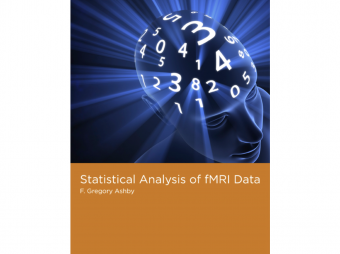# fMRI methods

Functional magnetic resonance imaging (fMRI), which allows researchers to observe neural activity in the human brain noninvasively, has revolutionized the scientific study of the mind. An fMRI experiment produces massive amounts of highly comples data; researchers face significant challenges in analyzing the data they collect. This book offers an overview of the most widely used statistical methods of analyzing fMRI data. Every step is covered, from preprocessing to advanced methods for assessing functional connectivity. The goal is not to describe which buttons to push in the popular software packages but to help readers understand the basic underlying logic, the assumptions, the strengths and weaknesses, and the appropriateness of each method.

The book covers all of the important current topics in fMRI data analysis, including the relation of the fMRI BOLD (blood oxygen-level dependent) response to neural activation; basic analyses done in virtually every fMRI article--preprocessing, constructing statistical parametrical maps using the general linear model, solving the multiple comparison problem, and group analyses; the most popular methods for assessing functional connectivity--coherence analysis and Granger causality; two widely used multivariate approches, principal components analysis and independent component anaylsis; and a brief survey of other current fMRI methods.

The neccessary mathematics is explained at a conceptual level, but in enough detail to allow mathematically sophisticated readers to gain more than a purely conceptual understanding. The book also includes short examples of Matlab code that implement many of the methods described; and appendix offers and introduction to basic Matlab matrix algebra commands (as well as a tutorial on matrix algebra). A second appendix introduces multivariate probability distributions.

Endorsements:

"Understanding the complexities associated with the generation of brain function images is essential, but this information is often difficult to obtain. This lovely new book by Ashby is a major step in meeting that need. I recommend it with enthusiasm to new initiates to imaging as well as seasoned veterans."

-Marcus Raichle, Professor of Radiology, Neurology, Neurobiology, and Biomedical Engineering at Washington University in St Louis

"This book covers all major fMRI analyses with a level of mathematical depth that is appropriate for cognitive and brain scientists with some background in statistics. The author has the rare ability of explaining complex issues using intuitive and engaging prose. The perfect balance between conceptual intelligibility and mathematical rigor makes this an ideal textbook for undergraduate and graduate courses."

-Roberto Cabeza, Professor of Psychology and Neuroscience, Duke University

Chapter 3: Modeling the BOLD Response

Box 3.1: Create and plot an hrf

Box 3.2: Convolve an hrf with a boxcar function to create a predicted BOLD response

Box 3.3: Generate BOLD predictions from the Volterra model

Chapter 4: Preprocessing

Box 4.1: The sinc interpolation of a difference-of-gammas hrf

Box 4.2: Coregistration using histogram bins

Box 4.3: The matched filter theorem

Box 4.4: High-pass temporal filtering

Chapter 5: The General Linear Model

Box 5.1: Generate a predicted BOLD vector for GLM analysis

Box 5.2: Present a design matrix visually

Box 5.3: Apply the correlation-based GLM to data

Chapter 6: The Multiple Comparisons Problem

Box 6.1: Implementing false discovery rate

Chapter 7: Group Analysis

Box 7.1: Fixed effects and random effects analysis of group data

Chapter 8: Coherence Analysis

Box 8.1: Compute and plot an autocorrelation function and a cross-correlation function

Box 8.2: Compute the power spectrum of a BOLD response

Box 8.3: Compute coherence between two BOLD responses

Box 8.4: Compute partial coherence between two BOLD responses without and with extra influence between them

Chapter 9: Granger Causality

Box 9.1: Create and fit autoregressive models of orders 1, 2, and 3

Box 9.2: Compute Granger causality Fx→y

Box 9.3: Compute conditional Granger causality Fi→j|k